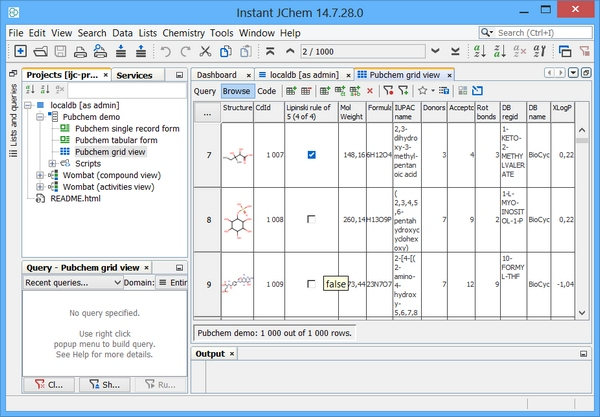# Triggers and sequences: Derby

1. Administer a table in Derby using Instant JChem.
2. Add a text field and populate it with a character based unique key.
3. Define an approach to derive a BOOLEAN data item "Lipinski rule of 5" for new rows based upon chemical terms, implemented as a "AFTER INSERT" trigger.
4. Define an approach to synchronise columns for which the source data is subsequently updated, implemented as a "AFTER UPDATE" trigger. This operation is required for example after a re-standardization operation is applied on a given JChem table.

It is hoped that these examples can be used as templates for further bespoke index based in Chemical terms or other calculated columns. In the case of Derby the "ij" tool can be used to connect to a schema but this requires some additional set up.

java org.apache.derby.tools.ij

Your local Derby database can be found in your windows home (Obviously for Linux this will be different). Once successful you should see the "ij>" command prompt.

`connect`` ``'jdbc:derby:C:\Users\Daniel\Documents\IJCProjects\ijc-project\.config\localdb\db'``;`

Alternatively the IJC database explorer will negate the need for this and connection using this approach for administration tasks is explained here: Using the database explorer

# 1. Administer Derby table using IJC

First, we need to administer a JChem table. One method to do this is via Instant JChem (IJC) Desktop application. Assuming we have an existing Derby connection / schema established in IJC then we can add a new JChem table easily. Right click on your schema and choose "new structure entity table". At the entity type drop down choose "New structure entity (using JChemBase table)". Assuming we accept the defaults - we should see the window below and the subsequent examples assume a table name of "STRUCTURES"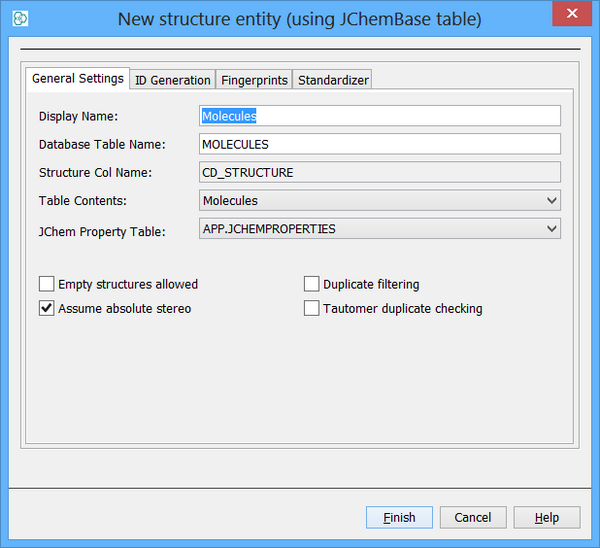# 2. Character based Unique key generation

A JChem table already has a build in unique integer key (CD_ID), however some organisations prefer to have a character based identifier since this can aid internal communication of structures. In order to create a character based sequence the integer based sequence is embedded in a trigger and prefixed with an appropriate string in this case "mol-" is used. If this is a "private" database then the Chemist initials (or lab notebook reference) might be suitable or perhaps a corporate identifier string is suitable if working with the central data repository.

An additional text based column is now required to store the character based key and this can be created using IJC. Right click on your table and choose "Edit data tree" Left click on "New standard field" and choose "Text field". You can also edit your display name and column name. In this case the column is named "TARGET_KEY". The Text field maps directly to a column of type VARCHAR in Derby. You also need to retain the "Required" value of FALSE.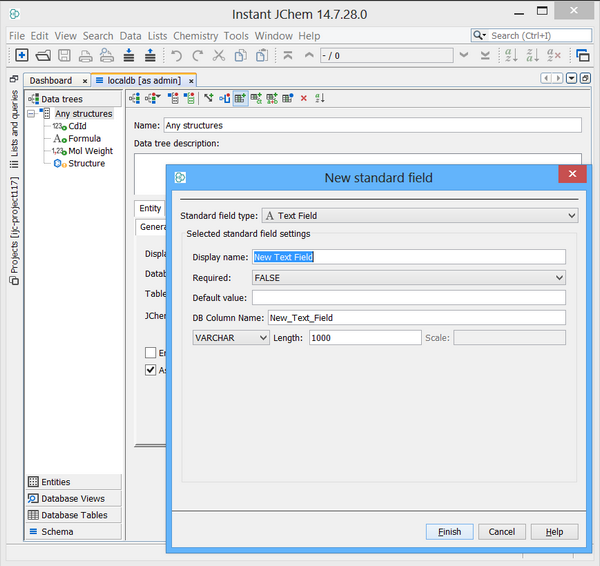Apache Derby RDBMS, now supports sequence objects, which can be used directly in our trigger definitions. First, at the ij prompt create a named sequence as in the code below.

`CREATE`` ``SEQUENCE`` StructuresSeq ``AS`` ``BIGINT`` START ``WITH`` 1 INCREMENT ``BY`` 1 MAXVALUE 1000000 ``NO`` CYCLE;`

We now create a trigger on the target table STRUCTURES that uses the sequence to populate the "TARGET_KEY" column.

Only one trigger is required to be defined. In Derby only a single SQL statement can be associated with each trigger but the triggers will fire in the order in which they are created.

`CREATE`` ``TRIGGER`` structures_key_instrg ``AFTER`` ``INSERT`` ``ON`` structures`
`REFERENCING NEW ``AS`` newrow ``FOR`` EACH ROW MODE DB2SQL ``UPDATE`` structures`
`SET`` target_key = ``'mol-'`` || ``CAST`` (``NEXT`` VALUE ``FOR`` StructuresSeq ``AS`` ``CHAR``(100)) ``WHERE`` target_key ``IS`` ``NULL``;`

To fire the trigger we should simply insert data into the table and for every row the action will occur. IJC will be completeing the insert (during data import) so you should not need to execute this syntax but your trigger will fire as a result of this action, if you should wish to test

`INSERT`` ``INTO`` structures (cd_id) ``values`` (1);`

# 3. Derived data item : Lipinski rule of 5 filter (New Inserts)

In order to create derivations from existing data an individual or set of triggers can be used. In this example a single drug like filter column "Lipinski_5" is created and populated for any rows that are added to a JChem table. The IJC boolean type actually maps to a SMALLINT type in Derby. You also need to retain the "Required" value of FALSE.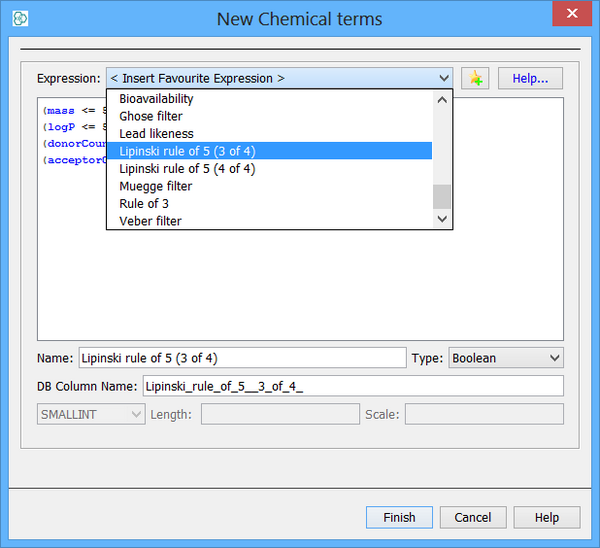A pre-requisite for this field is the existence of the necessary chemical terms columns it is derived from (at compile time). Also it is assumed they will be populated and not NULL and if created prior to a data import they will be populated. Several filter definitions already exist but the use of triggers provides an alternative option to avoid some repeat calculation and of course create new bespoke definitions. The Lipinski definition example here is taken from the following reference but has been slighty modified to include the value in each clause :

Reference : Chemoinformatics (Wiley), Page 607, Gasteiger J.

1. molecular weight <= 500 g / mol
2. a calculated log p <= 5
3. H-bond donors <= 5
4. H-bond acceptors <= 10
5. rotatable bond count <= 10

As such the following Chemical terms fields are selected for addition to the table and are populated on data import.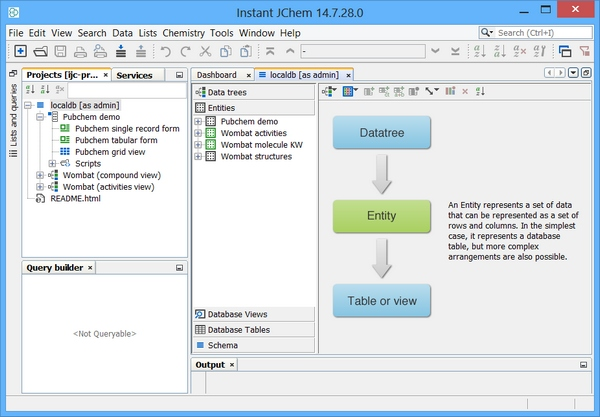Two triggers need to be defined order to implement this field. They should be created in the order below and will also be fired in that order

`CREATE`` ``TRIGGER`` structures_lipinski5_instrg1 ``AFTER`` ``INSERT`` ``ON`` structures`
`REFERENCING NEW ``AS`` newrow ``FOR`` EACH ROW MODE DB2SQL`
`UPDATE`` structures ``SET`` lipinksi_5 = 1`
`WHERE`` cd_molweight <= 500`
`AND`` logp <= 5`
`AND`` h_bond_donors <= 5`
`AND`` h_bond_acceptors <= 10`
`AND`` rotatable_bonds <= 10`
`AND`` lipinksi_5 ``is`` ``NULL`` `
`CREATE`` ``TRIGGER`` structures_lipinski5_instrg2`
`AFTER`` ``INSERT`` ``ON`` structures REFERENCING NEW ``AS`` newrow`
`FOR`` EACH ROW MODE DB2SQL ``UPDATE`` structures`
`SET`` lipinksi_5 = 0 ``WHERE`` lipinksi_5 ``is`` ``NULL``;`

# Derived data item: Lipinski rule of 5 filter (After table re-standartization or structure edit)

If the standardization rules for a given table are modified or a chemical structure edited then potentially the chemical terms might change also. Any index based on these columns will have no update mechanism defined. As such, one should also consider an after update trigger to re-calculate derived fields after a re-standardization event. Two triggers are required in order to implement as follows:

`CREATE`` ``TRIGGER`` structures_lipinski5_updtrg1 ``AFTER`` ``UPDATE`` ``ON`` structures`
`REFERENCING NEW ``AS`` newrow ``FOR`` EACH ROW MODE DB2SQL`
`UPDATE`` structures ``SET`` lipinksi_5 = 1`
`WHERE`` cd_molweight <= 500`
`AND`` logp <= 5`
`AND`` h_bond_donors <= 5`
`AND`` h_bond_acceptors <= 10`
`AND`` rotatable_bonds <= 10`
`AND`` lipinksi_5 ``is`` ``NULL``; `
`CREATE`` ``TRIGGER`` structures_lipinski5_updtrg2 ``AFTER`` ``UPDATE`` ``ON`` structures REFERENCING NEW ``AS`` newrow ``FOR`` EACH ROW MODE DB2SQL ``UPDATE`` structures ``SET`` lipinksi_5 = 0 ``WHERE`` lipinksi_5 ``is`` ``NULL``; `

The end result is a drug like filter and less time screening unneccessary molecules